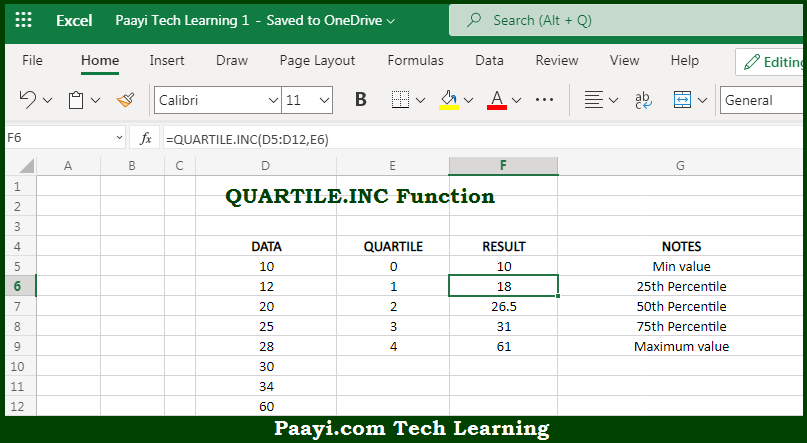# Learn How to Use Microsoft Excel QUARTILE.INC Function

Written by | 0 Comments | 563 Views

In this article, you will learn how to use Microsoft Excel QUARTILE.INC function and its prime function in Microsoft Excel. You will also get to know the Microsoft Excel QUARTILE.INC function return value and syntax with the help of some examples.

Microsoft Excel QUARTILE.INC Function

The main purpose of the Microsoft Excel QUARTILE.INC function is to get the quartile in the given data set. That implies, with the help of QUARTILE.INC function you can able to return the quartile of each of four equal groups for a given set of data. It should be noted that the QUARTILE.INC function can return minimum value, first quartile, second quartile, third quartile, and max value. Starting with Excel 2010, the QUARTILE.INC function replaces the QUARTILE function with the same behavior. So, with the help of QUARTILE.INC function, you can able to get the quartile in the given data set.

Return Value of QUARTILE.INC Function

The return value will be the value for the requested quartile.

Syntax of QUARTILE.INC Function

=QUARTILE.INC(array, quart)

Where the arguments:

• array: This is the reference containing data to analyze.
• quart: This is the quartile value to return.

## How to Use Microsoft Excel QUARTILE.INC Function?So we know that, Microsoft Excel QUARTILE.INC function you can able to get the quartile in the given data set. That implies, with the help of QUARTILE.INC function you can able to return the quartile of each of four equal groups for a given set of data. It should be noted that the QUARTILE.INC function can return minimum value, first quartile, second quartile, third quartile, and max value.

Starting with Excel 2010, the QUARTILE.INC function replaces the QUARTILE function with the same behavior. So, with the help of QUARTILE.INC function, you can able to get the quartile in the given data set.